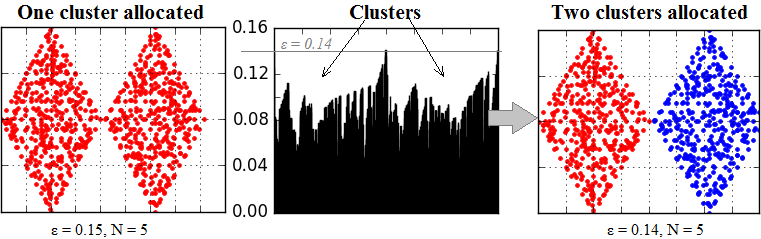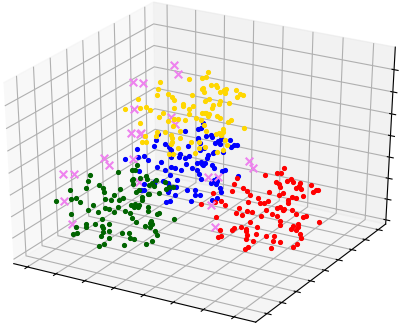pyclustering.cluster.optics.optics Class Reference

Class represents clustering algorithm OPTICS (Ordering Points To Identify Clustering Structure) with KD-tree optimization (ccore options is supported). More...

## Public Member Functions

def __init__ (self, sample, eps, minpts, amount_clusters=None, ccore=True, kwargs)
Constructor of clustering algorithm OPTICS. More...

def process (self)
Performs cluster analysis in line with rules of OPTICS algorithm. More...

def get_clusters (self)
Returns list of allocated clusters, where each cluster contains indexes of objects and each cluster is represented by list. More...

def get_noise (self)
Returns list of noise that contains indexes of objects that corresponds to input data. More...

def get_ordering (self)
Returns clustering ordering information about the input data set. More...

def get_optics_objects (self)
Returns OPTICS objects where each object contains information about index of point from processed data, core distance and reachability distance. More...

Returns connectivity radius that is calculated and used for clustering by the algorithm. More...

def get_cluster_encoding (self)
Returns clustering result representation type that indicate how clusters are encoded. More...

## Detailed Description

Class represents clustering algorithm OPTICS (Ordering Points To Identify Clustering Structure) with KD-tree optimization (ccore options is supported).

OPTICS is a density-based algorithm. Purpose of the algorithm is to provide explicit clusters, but create clustering-ordering representation of the input data. Clustering-ordering information contains information about internal structures of data set in terms of density and proper connectivity radius can be obtained for allocation required amount of clusters using this diagram. In case of usage additional input parameter 'amount of clusters' connectivity radius should be bigger than real - because it will be calculated by the algorithms if requested amount of clusters is not allocated.Scheme how does OPTICS works. At the beginning only one cluster is allocated, but two is requested. At the second step OPTICS calculates connectivity radius using cluster-ordering and performs final cluster allocation.

from pyclustering.cluster import cluster_visualizer
from pyclustering.cluster.optics import optics, ordering_analyser, ordering_visualizer
from pyclustering.samples.definitions import FCPS_SAMPLES
# Read sample for clustering from some file.
# Run cluster analysis where connectivity radius is bigger than real.
neighbors = 3
# Performs cluster analysis.
optics_instance.process()
# Obtain results of clustering.
clusters = optics_instance.get_clusters()
noise = optics_instance.get_noise()
ordering = optics_instance.get_ordering()
# Visualize clustering results.
visualizer = cluster_visualizer()
visualizer.append_clusters(clusters, sample)
visualizer.show()
# Display ordering.
analyser = ordering_analyser(ordering)
ordering_visualizer.show_ordering_diagram(analyser, 2)

Amount of clusters that should be allocated can be also specified. In this case connectivity radius should be greater than real, for example:

from pyclustering.cluster import cluster_visualizer
from pyclustering.cluster.optics import optics, ordering_analyser, ordering_visualizer
from pyclustering.samples.definitions import FCPS_SAMPLES
# Read sample for clustering from some file
# Run cluster analysis where connectivity radius is bigger than real
neighbors = 3
amount_of_clusters = 3
optics_instance = optics(sample, radius, neighbors, amount_of_clusters)
# Performs cluster analysis
optics_instance.process()
# Obtain results of clustering
clusters = optics_instance.get_clusters()
noise = optics_instance.get_noise()
ordering = optics_instance.get_ordering()
# Visualize ordering diagram
analyser = ordering_analyser(ordering)
ordering_visualizer.show_ordering_diagram(analyser, amount_of_clusters)
# Visualize clustering results
visualizer = cluster_visualizer()
visualizer.append_clusters(clusters, sample)
visualizer.show()

Here is an example where OPTICS extracts outliers from sample 'Tetra':

from pyclustering.cluster import cluster_visualizer
from pyclustering.cluster.optics import optics
from pyclustering.samples.definitions import FCPS_SAMPLES
# Read sample for clustering from some file.
# Run cluster analysis where connectivity radius is bigger than real.
neighbors = 3
# Performs cluster analysis.
optics_instance.process()
# Obtain results of clustering.
clusters = optics_instance.get_clusters()
noise = optics_instance.get_noise()
# Visualize clustering results (clusters and outliers).
visualizer = cluster_visualizer()
visualizer.append_clusters(clusters, sample)
visualizer.append_cluster(noise, sample, marker='x')
visualizer.show()

Visualization result of allocated clusters and outliers is presented on the image below:Clusters and outliers extracted by OPTICS algorithm from sample 'Tetra'.

Definition at line 284 of file optics.py.

## ◆ __init__()

 def pyclustering.cluster.optics.optics.__init__ ( self, sample, eps, minpts, amount_clusters = `None`, ccore = `True`, kwargs )

Constructor of clustering algorithm OPTICS.

Parameters
 [in] sample (list): Input data that is presented as a list of points (objects), where each point is represented by list or tuple. [in] eps (double): Connectivity radius between points, points may be connected if distance between them less than the radius. [in] minpts (uint): Minimum number of shared neighbors that is required for establishing links between points. [in] amount_clusters (uint): Optional parameter where amount of clusters that should be allocated is specified. In case of usage 'amount_clusters' connectivity radius can be greater than real, in other words, there is place for mistake in connectivity radius usage. [in] ccore (bool): if True than DLL CCORE (C++ solution) will be used for solving the problem. [in] **kwargs Arbitrary keyword arguments (available arguments: 'data_type').

Keyword Args:

• data_type (string): Data type of input sample 'data' that is processed by the algorithm ('points', 'distance_matrix').

Definition at line 395 of file optics.py.

## ◆ get_cluster_encoding()

 def pyclustering.cluster.optics.optics.get_cluster_encoding ( self )

Returns clustering result representation type that indicate how clusters are encoded.

Returns
(type_encoding) Clustering result representation.
get_clusters()

Definition at line 622 of file optics.py.

## ◆ get_clusters()

 def pyclustering.cluster.optics.optics.get_clusters ( self )

Returns list of allocated clusters, where each cluster contains indexes of objects and each cluster is represented by list.

Returns
(list) List of allocated clusters.
process()
get_noise()
get_ordering()

Definition at line 529 of file optics.py.

## ◆ get_noise()

 def pyclustering.cluster.optics.optics.get_noise ( self )

Returns list of noise that contains indexes of objects that corresponds to input data.

Returns
(list) List of allocated noise objects.
process()
get_clusters()
get_ordering()

Definition at line 545 of file optics.py.

## ◆ get_optics_objects()

 def pyclustering.cluster.optics.optics.get_optics_objects ( self )

Returns OPTICS objects where each object contains information about index of point from processed data, core distance and reachability distance.

Returns
(list) OPTICS objects.
get_ordering()
get_clusters()
get_noise()
optics_descriptor

Definition at line 588 of file optics.py.

## ◆ get_ordering()

 def pyclustering.cluster.optics.optics.get_ordering ( self )

Returns clustering ordering information about the input data set.

Clustering ordering of data-set contains the information about the internal clustering structure in line with connectivity radius.

Returns
(ordering_analyser) Analyser of clustering ordering.
process()
get_clusters()
get_noise()
get_optics_objects()

Definition at line 561 of file optics.py.

Referenced by pyclustering.cluster.optics.optics.process().

Returns connectivity radius that is calculated and used for clustering by the algorithm.

Connectivity radius may be changed only in case of usage additional parameter of the algorithm - amount of clusters for allocation.

Returns
get_ordering()
get_clusters()
get_noise()
get_optics_objects()

Definition at line 605 of file optics.py.

## ◆ process()

 def pyclustering.cluster.optics.optics.process ( self )

Performs cluster analysis in line with rules of OPTICS algorithm.

Returns
(optics) Returns itself (OPTICS instance).